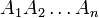# Kemperman's theorem

## Statement

### For two subsets

Suppose$G$ is a compact connected topological group with a Haar measure$\mu$ of total volume 1 (so that the Haar measure is a probability measure). Suppose$A,B$ are compact subsets of$G$. Then, the product of subsets$AB$ is also a compact subset and:$\! \mu(AB) \ge \min \{ \mu(A) + \mu(B), 1 \}$

### For finitely many subsets

Suppose$G$ is a compact connected topological group with a Haar measure$\mu$ of total volume 1 (so that the Haar measure is a probability measure). Suppose$A_1,A_2,\dots,A_n$ are compact subsets of$G$. Then, the product of subsets$A_1A_2\dots A_n$ is also a compact subset and:$\! \mu(A_1A_2 \dots A_n) \ge \min \{ \mu(A_1) + \mu(A_2) + \dots + \mu(A_n), 1 \}$

### Note for abelian groups

When,$G$ satisfies the additional condition of being an abelian group, and the group operations are denoted additively, the product of subsets is termed the Minkowski sum. Thus, this provides a lower bound on the size of the Minkowski sum.

## Caveats

### Importance of connectedness

Connectedness is important because if$G$ has a proper open subgroup, then by compactness it must have finite index, and then the measure of the subgroup is the reciprocal of that index. Taking$A,B$ to both be that subgroup violates the theorem.

### Sharpness of estimate

We can take$G$ to be the circle group and$A,B$ to be arcs starting at the identity element to show that the estimates cannot be improved.